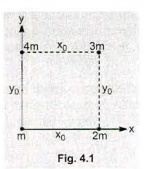# Problem: Four masses are arranged as shown in Fig. 4.1 Determine the x- and y-components of the gravitational force on the mass at the origin (m). Write the force in vector notation (i, j).

###### FREE Expert Solution

We'll use newton's law of gravity:

$\overline{){\mathbf{F}}{\mathbf{=}}\frac{\mathbf{G}{\mathbf{m}}_{\mathbf{1}}{\mathbf{m}}_{\mathbf{2}}}{{\mathbf{r}}^{\mathbf{2}}}}$

Gravitational force due to the 4m mass:

F4m = G(m)(4m)/(y0)2 = 4Gm2/y02 j

92% (83 ratings)###### Problem Details

Four masses are arranged as shown in Fig. 4.1 Determine the x- and y-components of the gravitational force on the mass at the origin (m). Write the force in vector notation (i, j).